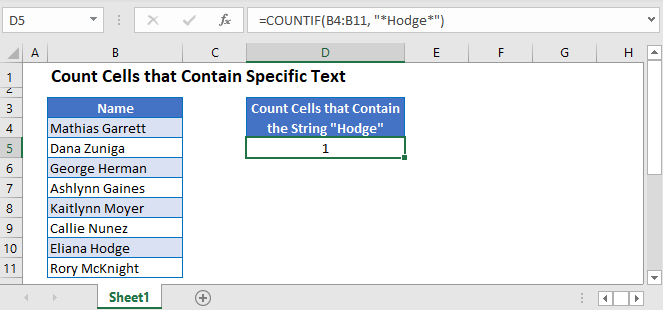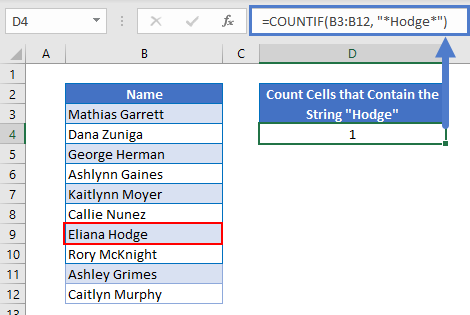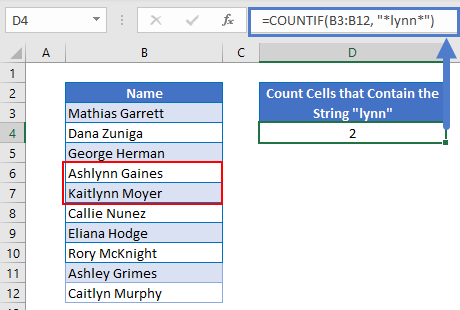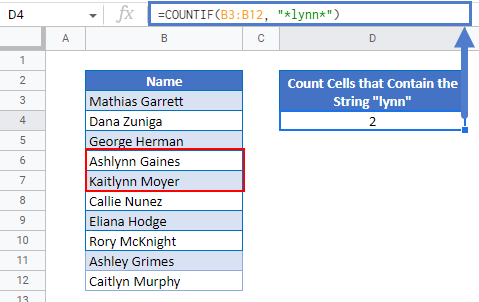# Count Cells that Contain Specific Text in Excel & Google Sheets

In this tutorial, we will demonstrate how to count cells that contain specific text in Excel and Google Sheets.## COUNTIF Function

The COUNTIF Function counts cells that meet a criteria. We can use the COUNTIF Function to count the number of cells that contain specific text by using asterisk wildcards.

Asterisk wildcards represent any number of any characters. By adding them before and after our text, we can count the number of cells where the text is found anywhere within the cell.

General Formula:

``=COUNTIF(Range, "*Text*")``

Example Formula:

``=COUNTIF(B3:B12, "*Hodge*")``By putting the last name “Hodge” inside of the asterisks, the COUNTIF Functions calculates all string values within the range that contain the name “Hodge”.

To demonstrate another example using the data set above, let’s say we wanted to find all the values in the range that contained the letters “lynn”.

We will use the following formula:

``=COUNTIF(B3:B12, "*lynn*")``We end up with two results in this case as A5 and A6 being Ashlynn Gaines and Kaitlynn Moyer as both have the letters “lynn” in their names.

Please note that the asterisk symbols are located inside of the quotation marks.

## Count Cells that Contain Specific Text in Google Sheets

The formula works exactly the same in Google Sheets as in Excel.### Excel Practice Worksheet

Practice Excel functions and formulas with our 100% free practice worksheets!